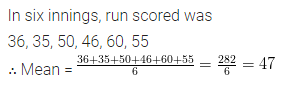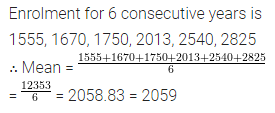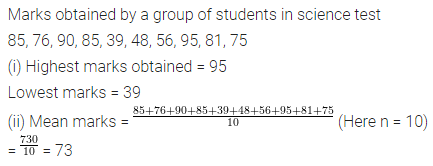ML Aggarwal Class 6 Solutions Chapter 15 Data Handling Ex 15.4 for ICSE Understanding Mathematics acts as the best resource during your learning and helps you score well in your exams.

## ML Aggarwal Class 6 Solutions for ICSE Maths Chapter 15 Data Handling Ex 15.4

Question 1.
Find the mean of the following data:
(i) 40, 30, 30, 0, 26, 60
(ii) 3, 5, 7, 9, 11, 13, 15
Solution:Question 2.
Find the mean of the first five even whole numbers.
Solution:Question 3.
A batsman scored the following number of runs in six innings:
36, 35, 50, 46, 60, 55
Calculate the mean runs scored by him in an inning.
Solution:Question 4.
The enrolment in a school for six consecutive years was as follows :
1555, 1670, 1750, 2013, 2540, 2825
Find the mean enrolment of the school for this period.
Solution:Question 5.
The marks (out of 100) obtained by a group of students in a science test are :
85, 76, 90, 85, 39, 48, 56, 95, 81, 75
Find the:
(i) highest and lowest marks obtained by the students.
(ii) mean marks obtained by the students.
Solution: